## # 定义

### # 流网络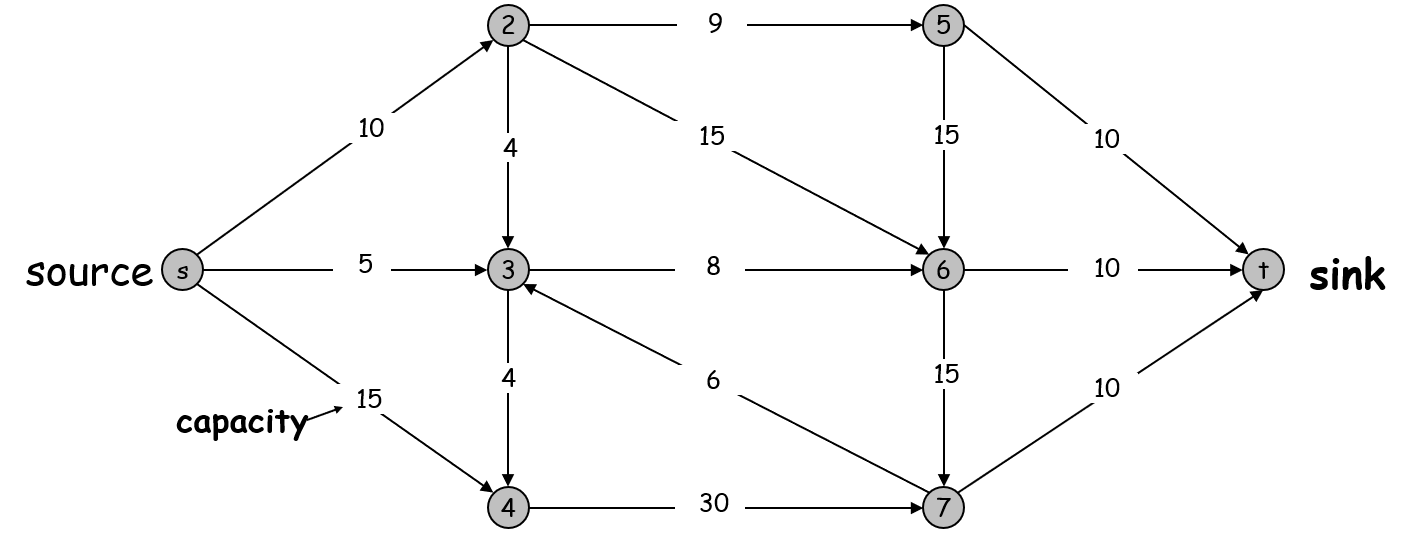1. 没有边进入 $s$ 点，没有点离开 $t$
2. 所有结点至少在一条边上
3. 所有的容量都为整数（这点很重要，是 Ford-Fulkerson 算法会结束的前提）

### # 流

$s-t$ 流是一个满足以下条件的函数：

1. 对于图中任意一边 $e$，有 $0 \leq f(e) \leq c(e)$
2. 对于图中任意一点 $v$，有 $\sum_\text{e in to v}f(e) = \sum_\text{e out of v}f(e)$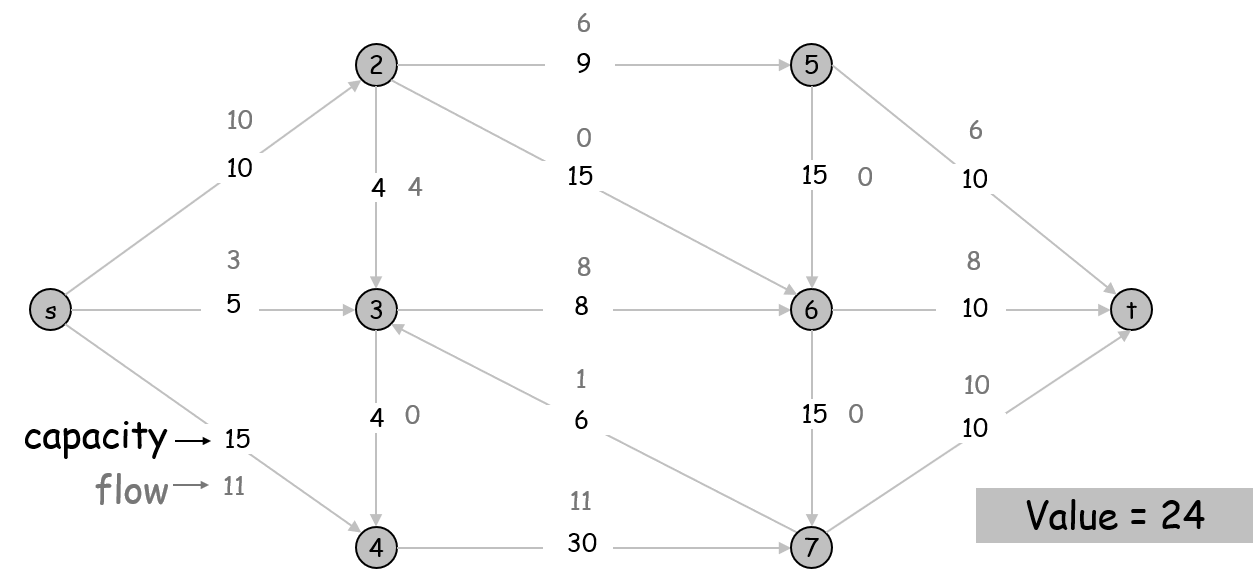### # 割

$s-t$ 割，就是把图分为 $A$$B$ 两块的一种方法，要满足 $A$ 块有 $s$$B$ 块有 $t$

$cap(A, B) = \sum_\text{e out of A} c(e)$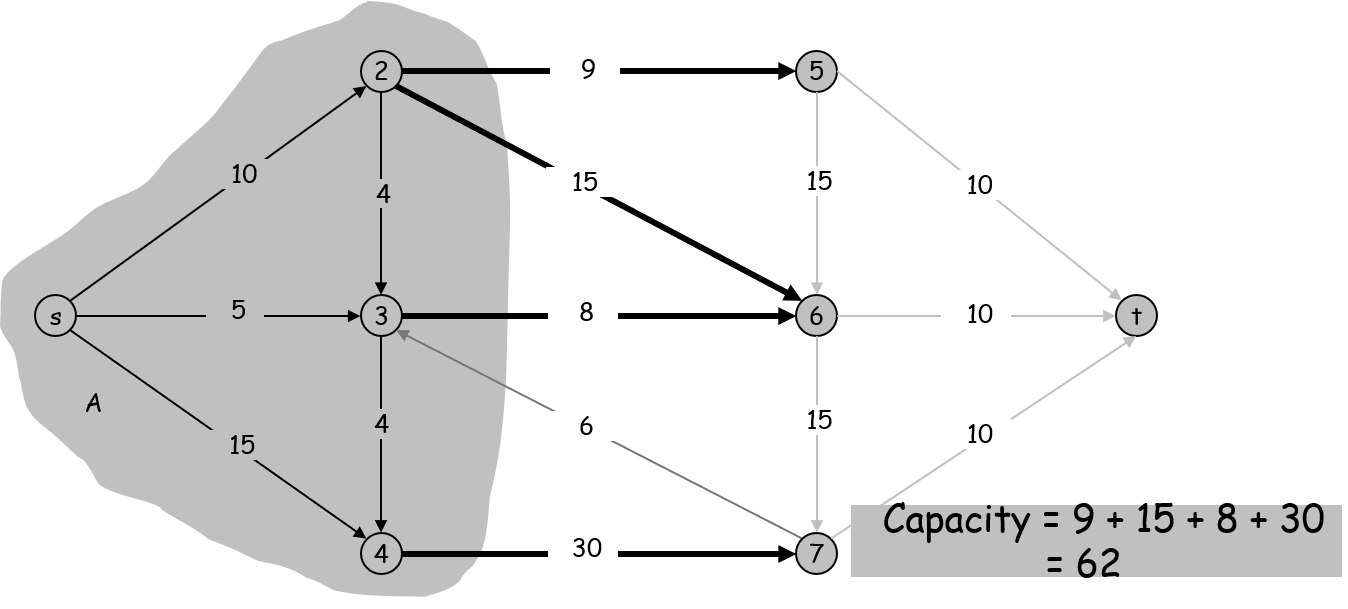## # 最大流-最小割定理

$\sum_\text{e out of A} {f(e)} - \sum_\text{e in to A} {f(e)} = v(f)$

\begin{aligned} v(f) &= \sum_\text{e out of s}f(e) & \text{(流的定义)} \\ &= \sum_{v \in A}\bigg(\sum_\text{e out of v}f(e) - \sum_\text{e in to v}f(e)\bigg) \qquad & \text{(加了一堆为值 0 的表达式)} \\ &= \sum_\text{e out of A}f(e) - \sum_\text{e in to A}f(e) & \text{(证明见后)}\\ \end{aligned}

• 如果某边 $e$ 的两个点都在 $A$ 内，这条边在第二行的贡献为一正一负，抵消为零；
• 如果某边 $e$ 穿出了 A（即入点在 $A$ 内，出点在 $B$ 内），这条边在第二行的贡献为一次正值；
• 如果某边 $e$ 穿入了 A（即入点在 $B$ 内，出点在 $A$ 内），这条边在第二行的贡献为一次负值。

$v(f) \leq cap(A,B)$

\begin{aligned} v(f) &= \sum_\text{e out of A}f(e) - \sum_\text{e in to A}f(e) \\ &\leq \sum_\text{e out of A}f(e) \\ &\leq \sum_\text{e out of A}c(e) \\ &=cap(A,B). \end{aligned}

## # 最大流算法

### # 贪心算法

1. 刚开始设所有边的 $f(e)=0$
2. 找到任意一条从 $s$ 连向 $t$，且每条边都还没有流满（即 $f(e) < c(e)$）的路径 P。增大该路径每条边上的流；
3. 反复执行上步操作直到流不动了。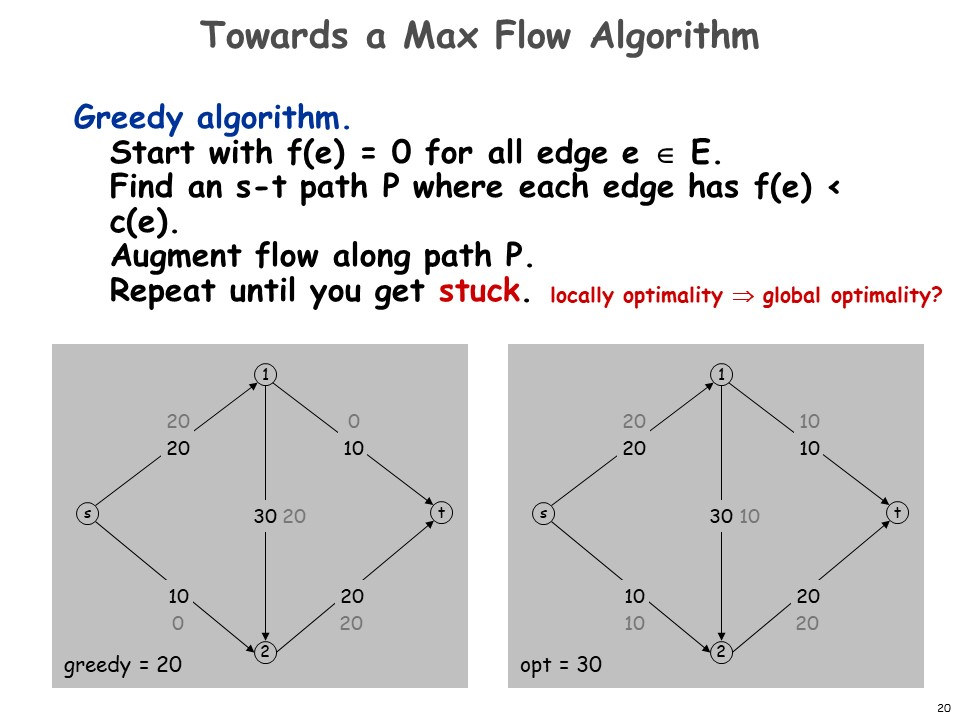### # 剩余图

$c_f(e)=\begin{cases} c(e) - f(e) & {\rm if} \quad e \in E \\ f(e) & {\rm if} \quad e^R \in E \end{cases}$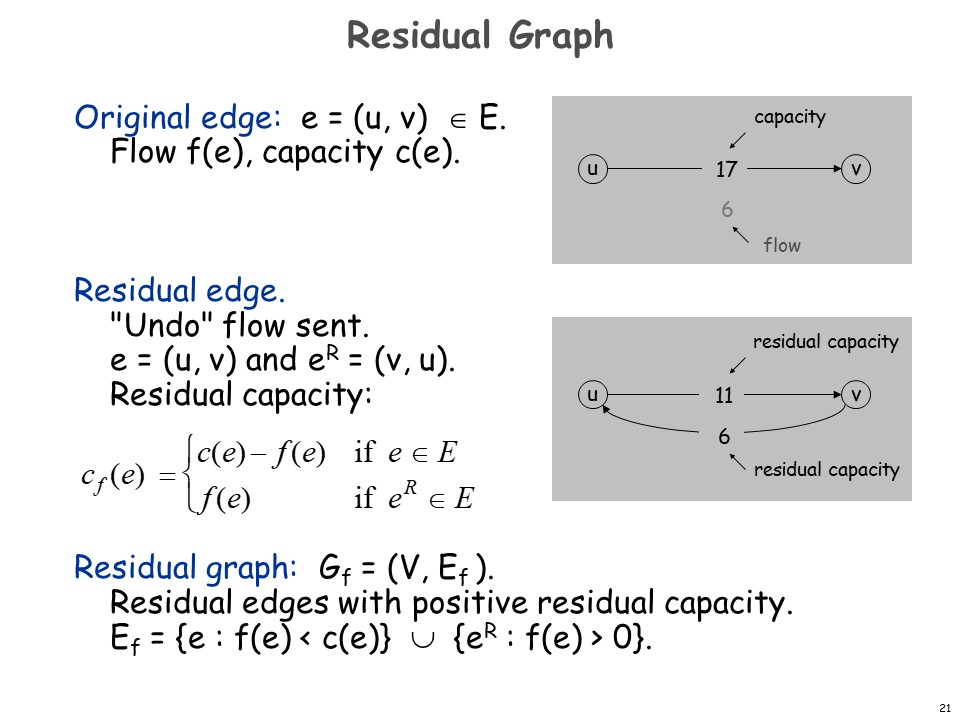### # Ford-Fulkerson 算法

Ford-Fulkerson 的算法即是先建立剩余图，然后在剩余图上进行我们最开始的贪心。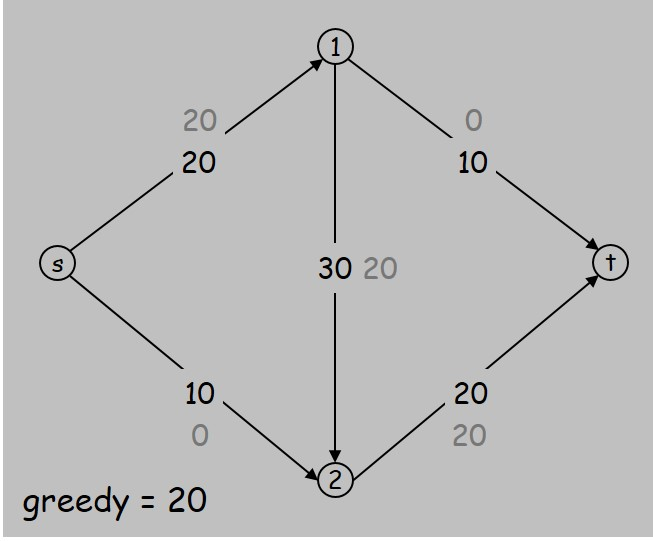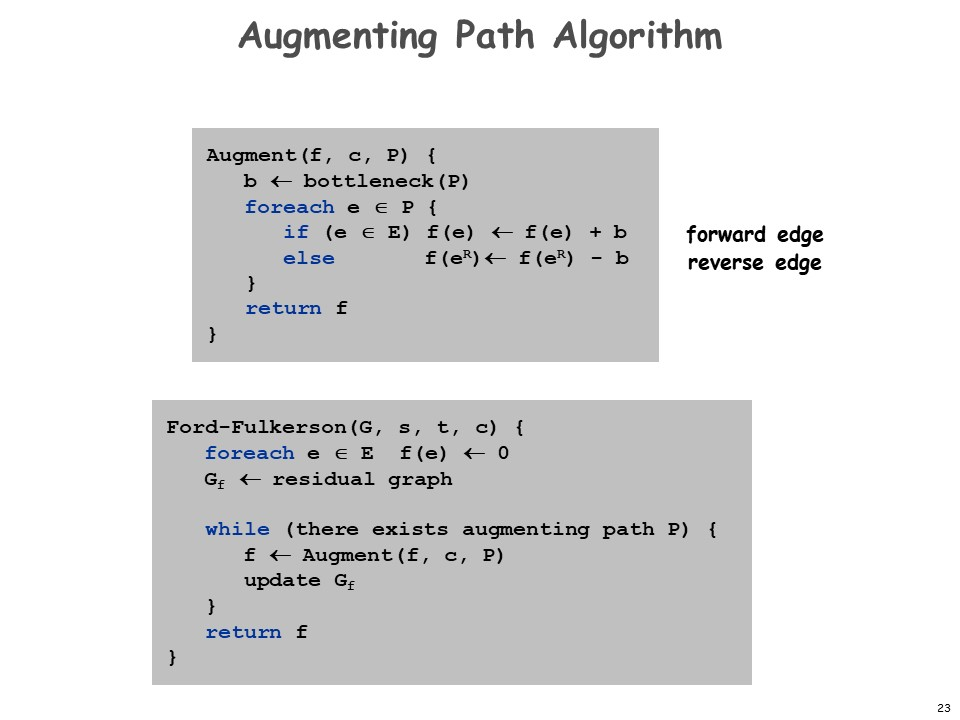#### # 最大流-最小割定理证明

(i) 存在割 $(A,B)$ 使得 $cap(A,B)=v(f)$
(ii) 流 $f$ 是最大流
(iii) 流 $f$ 不存在增广路

(i) -> (ii) 由前面的引理可得。

(ii) -> (iii) 反证：如果 $f$ 还存在增广路，按照 Ford-Fulkerson 的算法跑一下就可以使流的值变大，与最大流的前提矛盾。

(iii) -> (i) 设 $A$ 为在最后的剩余图从源点 $s$ 出发能到达的所有点的集合，$B=V-A$

\begin{aligned} v(f) &= \sum_\text{e out of A}f(e) - \sum_\text{e in to A} f(e) & \text{(由前面的引理)} \\ &= \sum_\text{e out of A}c(e) & \text{(证明见后)} \\ &= cap(A,B) & \text{(割容量的定义)} \end{aligned}

$e$ 在剩余图中仍然存在。
$e=(u,v)$，由 "out of" 的定义， $u \in A$$v \notin A$。 由 $A$ 的假设，在剩余图中 $s$$u$ 存在通路。 将该通路与 $e$ 拼接，则 $s$$v$ 也有通路，与 $v \notin A$ 矛盾。

#### # 时间复杂度

Ford-Fulkerson 算法会停止的证明中，我们证明了

• $v(f)$ 有一个上界为 $\sum_\text{e out of s}c(e)$，也就是 $O(mC)$
• 算法每次迭代必定增长 1。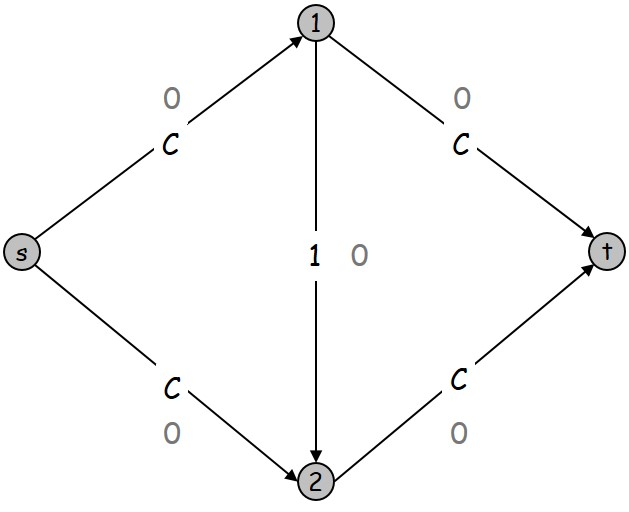### # 缩放最大流算法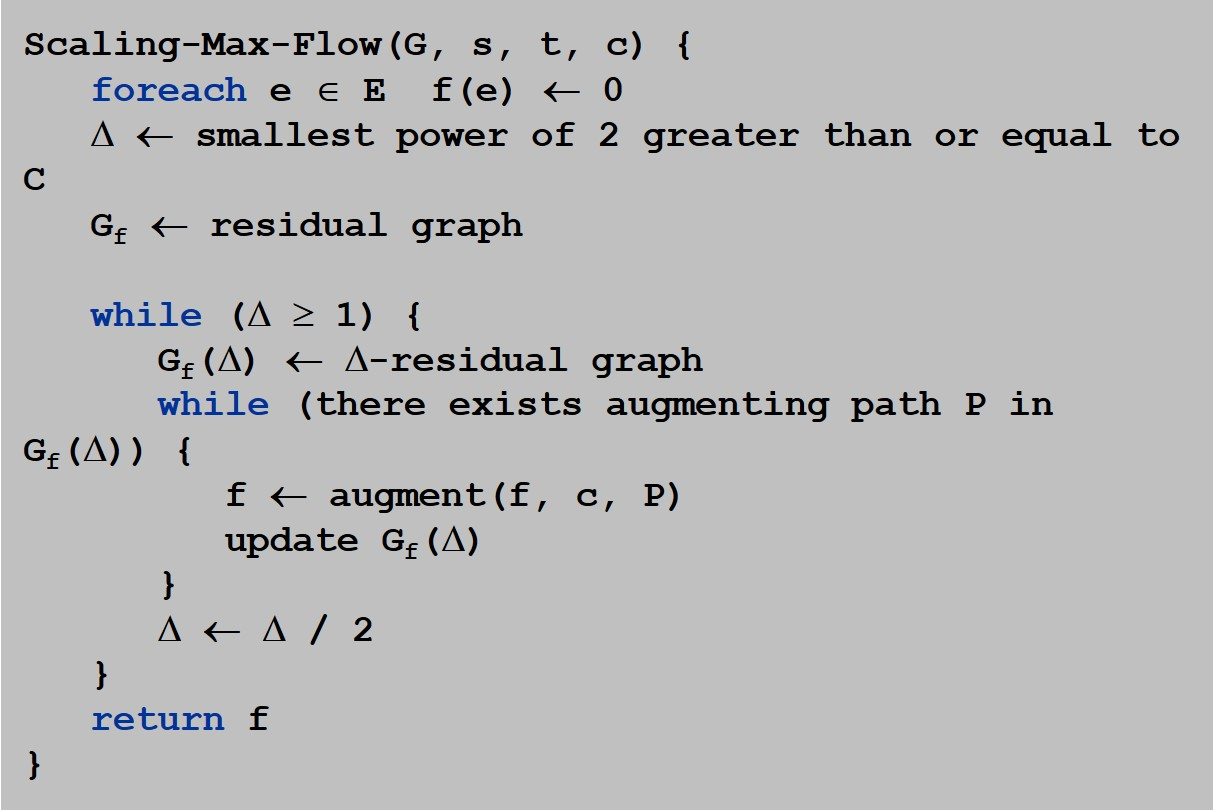Dinitz、Edmonds 和 Karp 分别独立证明了增广路算法能够在